# How to insert an equation with matrix

Word
This tip displays how to add an equation with matrix, for example, the Electromagnetic tensor.

Do the following (how to add an equation in your document, see Working with Microsoft Equation):

## In the Professional format:

2.   On the Equation tab, in the Structures group, click the Script button: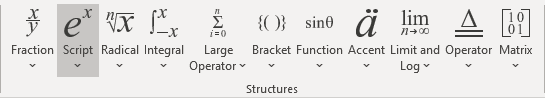In the Script list, choose Subscript: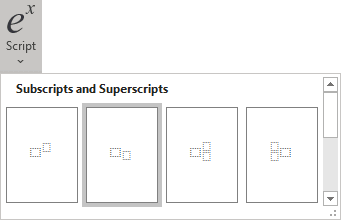3.   In the base box of the script, enter F, and then in the lower right box of the script:

• On the Equation tab, in the Symbols group, click the More button: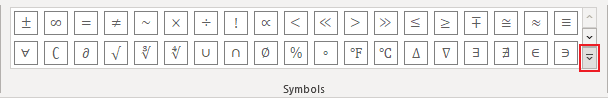• In the list of symbols, choose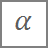and then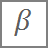.

4.   Enter =.

5.   On the Equation tab, in the Structures group, click the Bracket button. In the Brackets list, choose round brackets: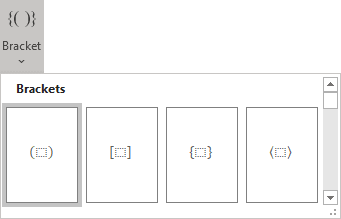6.   On the Equation tab, in the Structures group, click the Matrix button. In the Empty Matrices list, choose 2x2 Empty Matrix or 3x3 Empty Matrix: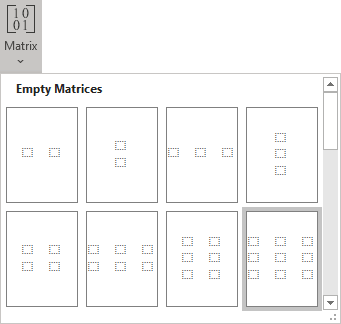7.   To increase a count of columns or/and rows of your matrix:

• right-click in it,
• in the Insert list of the popup menu, choose what and how you want to increase: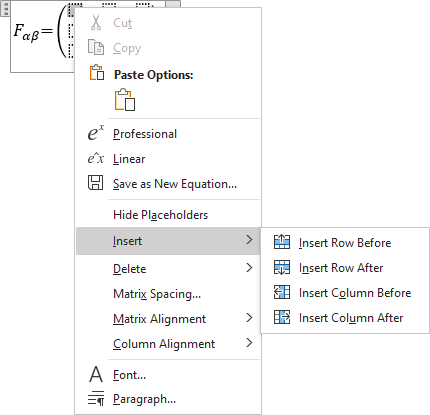8.   Enter in some positions zero, for other positions use Subscript and Fraction: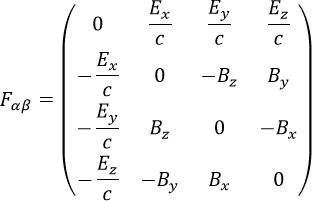## In the Linear format:

1.   In your own equation, enter F_.

2.   On the Equation tab, in the Symbols group, choose(or \alpha) and then(or \beta).

3.   Enter =. Then you enter a symbol equal, this linear formula transformed to the professional format: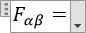4.   In the brackets, enter the following:

(\matrix(0&E_x/c&E_y/c&E_z/[email protected](-E_x)/c&0&-B_z&[email protected](-E_y)/c&B_z&0&[email protected](-E_z)/c&-B_y&B_x&0));

where \matrix is a command to create a matrix, & divides elements to columns in one row, @ divides rows in the matrix. Every time then you enter a special symbol, this linear formula transformed into a professional format.

Note: You can change spaces and alignment of your matrix, for more details see Adjusting spacing and alignment in an equation.